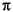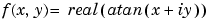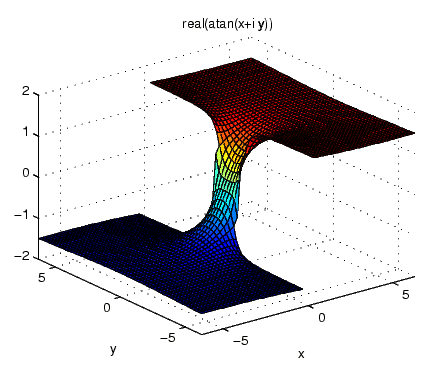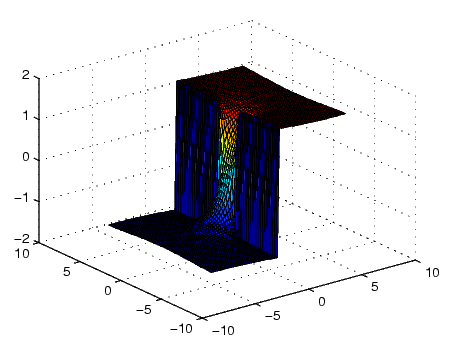MATLAB Function Referenceezsurf

Easy to use 3-D colored surface plotter

Syntax

• ```ezsurf(fun)
ezsurf(fun,domain)
ezsurf(funx,funy,funz)
ezsurf(funx,funy,funz,[smin,smax,tmin,tmax]) or
ezsurf(funx,funy,funz,[min,max])
ezsurf(...,n)
ezsurf(...,'circ')
ezsurf(axes_handle,...)
h = ezsurf(...)
```

Description

```ezsurf(fun) ``` creates a graph of `fun(x,y)` using the `surf` function. `fun` is plotted over the default domain: -2< `x` < 2, -2< `y` < 2.

`fun` can be a function handle for an M-file function or an anonymous function (see Function Handles and Anonymous Functions) or a string (see the Remarks section).

```ezsurf(fun,domain) ``` plots `fun` over the specified `domain`. `domain` can be either a 4-by-1 vector [`xmin`, `xmax`, `ymin`, `ymax`] or a 2-by-1 vector [`min`, `max`] (where `min` < `x` < `max`, `min` < `y` < `max`).

```ezsurf(funx,funy,funz) ``` plots the parametric surface `funx(s,t)`, `funy(s,t)`, and f`unz(s,t)` over the square: -2< `s` < 2, -2< `t` < 2.

```ezsurf(funx,funy,funz,[smin,smax,tmin,tmax]) or ezsurf(funx,funy,funz,[min,max]) ``` plots the parametric surface using the specified domain.

```ezsurf(...,n) ``` plots `fun` over the default domain using an `n`-by-`n` grid. The default value for `n` is 60.

```ezsurf(...,'circ') ``` plots `fun` over a disk centered on the domain.

```ezsurf(axes_handle,...) ``` plots into the axes with handle `axes_handle` instead of the current axes (`gca`).

```h = ezsurf(...) ``` returns the handle to a surface object in `h`.

Remarks

Passing the Function as a String

Array multiplication, division, and exponentiation are always implied in the expression you pass to `ezmesh`. For example, the MATLAB syntax for a surface plot of the expression

• ```sqrt(x.^2 + y.^2);
```

is written as

• ```ezsurf('sqrt(x^2 + y^2)')
```

That is, `x^2` is interpreted as `x.^2` in the string you pass to `ezsurf`.

If the function to be plotted is a function of the variables u and v (rather than x and y), then the domain endpoints `umin`, `umax`, `vmin`, and `vmax` are sorted alphabetically. Thus, `ezsurf('u^2 - v^3',[0,1],[3,6])` plots u2 - v3 over 0 < u < 1, 3 < v < 6.

Passing a Function Handle

Function handle arguments must point to functions that use MATLAB syntax. For example, the following statements define an anonymous function and pass the function handle `fh` to `ezsurf`.

• ```fh = @(x,y) sqrt(x.^2 + y.^2);
ezsurf(fh)
```

Note that when using function handles, you must use the array power, array multiplication, and array division operators (`.^, .*, ./`) since `ezsurf` does not alter the syntax, as in the case with string inputs.

Passing Additional Arguments

If your function has additional parameters, for example `k` in `myfun`:

• ```function z = myfun(x,y,k1,k2,k3)
z = x.*(y.^k1)./(x.^k2 + y.^k3);
```

then you can use an anonymous function to specify that parameter:

• ```ezsurf(@(x,y)myfun(x,y,2,2,4))
```

Examples

`ezsurf` does not graph points where the mathematical function is not defined (these data points are set to `NaN`s, which MATLAB does not plot). This example illustrates this filtering of singularities/discontinuous points by graphing the function

•over the default domain -2< x < 2, -2< y < 2:

• ```ezsurf('real(atan(x+i*y))')```

Using `surf` to plot the same data produces a graph without filtering of discontinuities (as well as requiring more steps):

• ```[x,y] = meshgrid(linspace(-2*pi,2*pi,60));
z = real(atan(x+i.*y));
surf(x,y,z)```

Note also that `ezsurf` creates graphs that have axis labels, a title, and extend to the axis limits.

See Also

`ezmesh`, `ezsurfc`, `function_handle`, `surf`

Function Plots for related functions

© 1994-2005 The MathWorks, Inc.